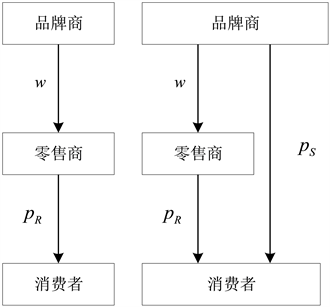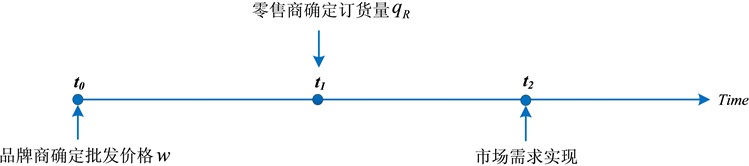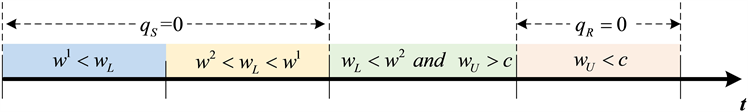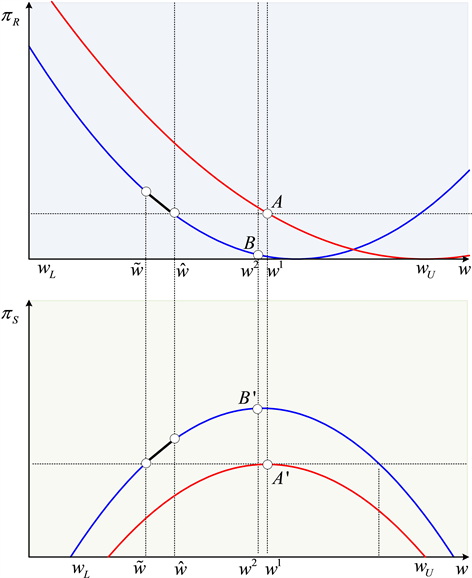#### 期刊菜单

Supplier Encroachment Strategies Based on Wholesale Price Commitment
DOI: 10.12677/MSE.2022.114064, PDF , HTML, XML, 下载: 86  浏览: 152

Abstract: With the continuous development of Chinese online shopping economy, more and more brands have encroached the retail channel by establishing direct channels. This paper studies the Encroachment decision of brands when they can credibly commit to the wholesale price with the existence of product experience. Firstly, the equilibrium decisions of brand in single-channel and dual-channel are analyzed, and then the effects of product experience and wholesale price commitment on brand encroachment strategy and supply chain profit are explored. It is found that when the product experience is medium high, the sales volume and profit of dual-channel sales can be better than that of single-channel case by the brand owner credibly promising a lower wholesale price which the retailer can predict the decision of the brand in advance, so the retailer is willing to accept the brand establishing the direct channel and realize channel coordination.

1. 引言

2. 文献综述

3. 模型说明Figure 1. The supply chain structure

4. 单渠道销售时供应链成员的均衡决策

4.1. 单渠道销售的博弈时序分析Figure 2. Game sequence

4.2. 单渠道销售的均衡结果分析

${U}^{1}\left(u,p;\theta \right)>0$，即 $\theta \ge \frac{{p}_{R}}{u}$ 时，消费者才愿意购买该产品。不失一般性，将市场标准化为1，需求相应为 ${q}_{R}=1-\frac{{p}_{R}}{u}$。因此，得到了逆需求函数为： ${p}_{R}=u\left(1-{q}_{R}\right)$

${\pi }_{R}=\left({p}_{R}-w\right){q}_{R}$ (1)

${\pi }_{S}=\left(w-c\right){q}_{R}$ (2)

${w}^{1}=\frac{c+u}{2}$ (3)

${q}_{R}^{1}=\frac{-c+u}{4u}$ (4)

${p}_{R}^{1}=\frac{c+3u}{4}$ (5)

${\pi }_{S}^{1}=\frac{{\left(c-u\right)}^{2}}{8u}$ (6)

${\pi }_{R}^{1}=\frac{{\left(c-u\right)}^{2}}{16u}$ (7)

5. 双渠道销售时供应链成员的均衡决策

5.1. 品牌商侵入后博弈时序分析Figure 3. The game sequence in dual-channel supply chain

5.2. 品牌商侵入后均衡结果分析

${p}_{S}=tu\left(1-{q}_{R}-{q}_{S}\right)$ (8)

${p}_{R}=u\left(1-{q}_{R}-t{q}_{S}\right)$ (9)

${\pi }_{S}^{2}=\left(w-c\right){q}_{R}+\left({p}_{S}-c\right){q}_{S}-F$ (10)

${\pi }_{R}^{2}=\left({p}_{R}-w\right){q}_{R}$ (11)

${q}_{R}^{2}\left({q}_{S}^{2},w\right)=\frac{u-tu{q}_{S}-w}{2u}$ (12)

${q}_{S}^{2}\left({q}_{R}^{2},w\right)=\frac{-c+tu-tu{q}_{R}}{2tu}$ (13)

${q}_{R}^{2}\left(w\right)=\frac{-c+\left(-2+t\right)u+2w}{\left(-4+t\right)u}$ (14)

${q}_{S}^{2}\left(w\right)=\frac{2c-t\left(u+w\right)}{\left(-4+t\right)tu}$ (15)

${w}^{2}=\frac{1}{2}\left(c-\frac{\left(8+\left(-4+t\right)t\right)u}{-8+3t}\right)$ (16)

${q}_{R}^{2}=\frac{2\left(-1+t\right)}{-8+3t}$ (17)

${q}_{S}^{2}=\frac{1}{2}\left(\frac{-6+t}{-8+3t}-\frac{c}{tu}\right)$ (18)

${p}_{R}^{2}=\frac{1}{2}\left(c-\frac{\left(-6+t\right)\left(-2+t\right)u}{-8+3t}\right)$ (19)

${p}_{S}^{2}=\frac{1}{2}\left(c+\frac{\left(-6+t\right)tu}{-8+3t}\right)$ (20)

${\pi }_{R}^{2}=\frac{4{\left(-1+t\right)}^{2}u}{{\left(8-3t\right)}^{2}}$ (21)

${\pi }_{s}^{2}=\frac{1}{4}\left(-2c+\frac{{c}^{2}}{tu}-\frac{\left(4+{t}^{2}\right)u}{-8+3t}\right)$ (22)

5.3. 产品体验性对品牌商侵入的影响

$w<{w}_{U}=\frac{1}{2}\left(c-\left(-2+t\right)u\right)$

$w>{w}_{L}=\frac{2c}{t}-u$

$c

a) 低的 $t\left(t<\frac{4c}{c+3u}\right)$ ：此时，产品的体验性非常高，品牌商通过直销渠道销售产品不能够满足此类产品的体验需求，因此线上销售不具有竞争力，即使品牌商开通直销渠道，消费者依然只从零售渠道购买产品( ${q}_{R}^{2}>0$${q}_{S}^{2}=0$ )。

b) 中等低的 $t\left(\frac{4c}{c+3u} ：品牌商开通直销渠道的同时，也降低了批发价格，这使得零售商有足够空间降低其零售价 ${p}_{S}^{2}$，直到使直销渠道均衡需求下降至零，即消费者只购买零售渠道产品( ${q}_{R}^{2}>0$${q}_{S}^{2}=0$ )。

1) 品牌商将降低产品的批发价格至 ${w}_{L}<{w}^{1}$，此批发价折扣( ${w}^{1}-{w}_{L}=\frac{t\left(c+2u\right)-4c}{2t}$ )随着产品体验性t的增加而增加，随着生产成本c的增加而减少；

2) 即使零售商降低零售价( ${p}_{R}^{2}\left({w}_{L}\right)<{p}_{R}^{1}$ )，其边际利润仍然得到提高，即 ${p}_{R}^{2}\left({w}_{L}\right)-{w}_{L}>{p}_{R}^{1}-{w}^{1}$

c) 中等高的 $t\left(\frac{3c+6u-\sqrt{9{c}^{2}+4cu+36{u}^{2}}}{2u} ：如果品牌商开通直销渠道，在均衡状态下，两个销售渠道可以同时存在，消费者将根据自身需求选择渠道购买产品( ${q}_{R}^{2}>0$${q}_{S}^{2}>0$ )。

1) 品牌商降低零售渠道的批发价格至 ${w}^{2}<{w}^{1}$，此时批发价折扣( ${w}^{1}-{w}^{2}=\frac{\left(-1+t\right)tu}{-16+6t}$ )随着产品体验性t先增后减；

2) 当 $t\in \left(\frac{8c}{3c+5u},2-\frac{c}{u}\right)$，即使零售商降低批发零售价格( ${p}_{R}^{2}<{p}_{R}^{1}$ )，其边际利润仍然得到提高( ${p}_{R}^{2}-{w}^{2}>{p}_{R}^{1}-{w}^{1}$ )。

3) 品牌商从零售渠道获得的边际利润总是大于直销渠道的边际利润( ${w}^{2}>{p}_{S}^{2}$ )。

d) 较高的 $t\left(t>2-\frac{c}{u}\right)$ ：此时产品体验性非常低，消费者对于购买渠道没有要求，且较低的线上零售价格，使线下零售渠道需求下降至0，零售商退出市场( ${q}_{R}^{2}=0$${q}_{S}^{2}>0$ )。Figure 4. The effect of product experience t

1) 总销售量增加( ${q}_{R}^{2}+{q}_{S}^{2}>{q}_{R}^{1}$ )；

2) 零售商订货量可能增加( ${q}_{R}^{2}>{q}_{R}^{1}↔t>\frac{8c}{3c+5u}$ )。

5.4. 批发价承诺对品牌商侵入的影响

1) 当品牌商不能够承诺批发价格时

${F}^{nc}\equiv {\pi }_{S}^{2}\left(w={w}^{2}\right)-{\pi }_{S}^{1}\left(w={w}^{1}\right)$ (23)

2) 当品牌商可以承诺批发价时

${\pi }_{R}^{2}\left(w=\stackrel{^}{w}\right)={\pi }_{R}^{1}\left(w=\stackrel{^}{w}\right)$ (24)

${\pi }_{S}^{2}\left(w=\stackrel{˜}{w}\right)-F={\pi }_{S}^{1}\left(w={w}^{1}\right)$ (25)

$\begin{array}{c}\stackrel{˜}{w}=\frac{c}{2}+\frac{1}{4\left(-8+3t\right)}u\left(t\left(8-8\sqrt{2}\sqrt{\frac{{c}^{2}\left(16-14t+3{t}^{2}\right)+2ct\left(-8+3t\right)u+tu\left(8F\left(-8+3t\right)+t\left(3+2t\right)u\right)}{{\left(-4+t\right)}^{2}t{u}^{2}}}\right)\\ \text{\hspace{0.17em}}+{t}^{2}\left(-2+\sqrt{2}\sqrt{\frac{{c}^{2}\left(16-14t+3{t}^{2}\right)+2ct\left(-8+3t\right)u+tu\left(8F\left(-8+3t\right)+t\left(3+2t\right)u\right)}{{\left(-4+t\right)}^{2}t{u}^{2}}}\right)\\ \text{\hspace{0.17em}}+16\left(-1+\sqrt{2}\sqrt{\frac{{c}^{2}\left(16-14t+3{t}^{2}\right)+2ct\left(-8+3t\right)u+tu\left(8F\left(-8+3t\right)+t\left(3+2t\right)u\right)}{{\left(-4+t\right)}^{2}t{u}^{2}}}\right)\right)\end{array}$

${F}^{x}\equiv {\pi }_{S}^{2}\left(w={w}^{2}\right)-{\pi }_{S}^{1}\left(w={w}^{2}\right)$ (26)

${F}^{x}=\frac{1}{8}\left(-2c-\frac{{c}^{2}\left(-2+t\right)}{tu}+\frac{\left(-6+t\right)t\left(-4+\left(-2+t\right)t\right)u}{{\left(8-3t\right)}^{2}}\right)$Figure 5. The profit of the brand owner and the retailer

${w}^{1}$ 表示单一渠道销售的最优批发价， ${w}^{2}$ 表示双渠道的最优批发价。观察图3~5可以看出，当开通双渠道后，零售商的利润从A点降到了B点。通过前文分析可知，当零售商因为利润下降而退出与品牌商的合作时，也会损害品牌商的利益。为避免此类情况，品牌商愿意承诺一个比均衡批发价更低的价格，从而不损害零售商利润，也就是使零售商的利润要高于图中点A的位置。因此，零售商期望的批发价格应不超过 $\stackrel{^}{w}$。而对于品牌商而言，开设双渠道后能够获得的利润不低于单一渠道销售时的利润A'点，就是有利可图的。因此，批发价的最低值不应低于 $\stackrel{˜}{w}$，此时品牌商的利润等于在传统零售渠道能够获得的利润。因此，综合上述分析，品牌商的最优批发价应在 $\left(\stackrel{˜}{w},\stackrel{^}{w}\right)$ 之间，此时双方均实现利润的增长，开通线上直销渠道是一个双赢的合作策略。

6. 结论

  中国互联网络信息中心. 第50次《中国互联网络发展状况统计报告》[EB/OL]. http://www.cnnic.net.cn/n4/2022/0914/c88-10226.html, 2022-08-31.  Arya, A., Mittendorf, B. and Sappington, D. (2007) The Bright Side of Supplier Encroachment. Marketing Science, 26, 651-659. https://doi.org/10.1287/mksc.1070.0280  Xiong, Y., Yan, W., Fernandes, K., et al. (2012) “Bricks vs. Clicks”: The Impact of Manufacturer Encroachment with a Dealer Leasing and Selling of Durable Goods. European Journal of Operational Research, 217, 75-83. https://doi.org/10.1016/j.ejor.2011.08.012  Cai, G.G. (2010) Channel Selection and Coordination in Dual-Channel Supply Chains. Journal of Retailing, 86, 22-36. https://doi.org/10.1016/j.jretai.2009.11.002  孙自来, 王旭坪, 阮俊虎, 樊文平. 不同权力结构对制造商双渠道供应链的影响研究[J]. 运筹与管理, 2020, 29(9): 106-114.  周建亨, 赵瑞娟. 搭便车效应影响下双渠道供应链信息披露策略[J]. 系统工程理论与实践, 2016, 36(11): 2839-2852.  Matsui, K. (2017) When Should a Manufacturer Set Its Direct Price and Wholesale Price in Dual-Channel Supply Chains? European Journal of Operational Research, 258, 501-511. https://doi.org/10.1016/j.ejor.2016.08.048  李波, 王汝锋, 陈蔚淳. 电子商务下风险规避制造商对供应链决策策略的影响研究[J]. 管理工程学报, 2019, 33(2): 173-179.  Meryer, C. and Schwager, A. (2007) Understanding Customer Experience. Harvard Business Review, No. 2, 1-15.  Dumrongsiri, A., Fan, M., Jain, A., et al. (2008) A Supply Chain Model with Direct and Retail Channels. European Journal of Operational Research, 187, 691-718. https://doi.org/10.1016/j.ejor.2006.05.044  宋巍. B2C电子商务顾客体验对顾客忠诚度的影响研究[D]: [硕士学位论文]. 济南: 山东大学, 2012: 1-10.  Groznik, A. and Heese, H.S. (2010) Supply Chain Conflict due to Store Brands: The Value of Wholesale Price Commitment in a Retail Supply Chain. Decision Sciences, 41, 203-230. https://doi.org/10.1111/j.1540-5915.2010.00265.x  Su, X. and Zhang, F. (2008) Strategic Customer Behavior, Commitment, and Supply Chain Performance. Management Science, 54, 1759-1773. https://doi.org/10.1287/mnsc.1080.0886  Hu, H., Sun, S., Zheng, X., et al. (2021) Committed or Contingent? The Retailer’s Information Acquisition and Sharing Strategies When Confronting Manufacturer Encroachment. International Journal of Production Economics, 242, Article ID: 108294.